# balunCoupledLine

Create multisection coupled-line balun on X-Y plane

Since R2022a

## Description

Use the `balunCoupledLine` object to create a multisection coupled-line balun with an unbalanced input and a balanced output. The output signal has a phase difference of 180 degrees.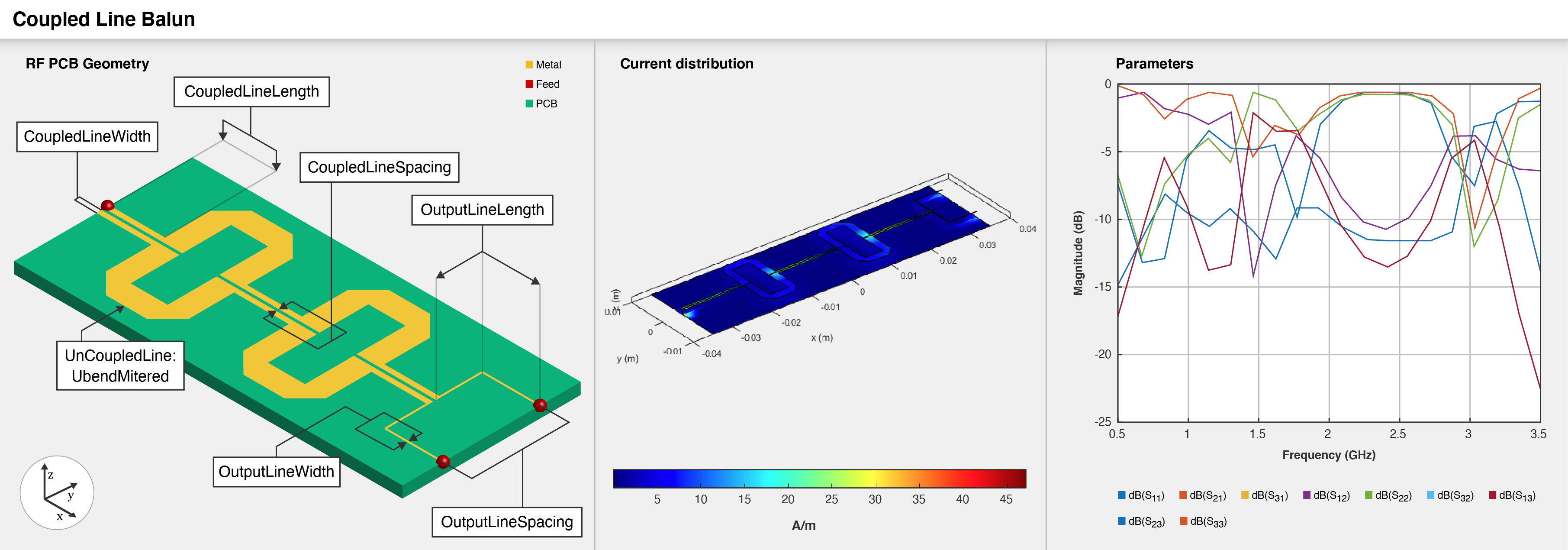## Creation

### Syntax

``balun = balunCoupledLine``
``balun = balunCoupledLine(Name=Value)``

### Description

example

````balun = balunCoupledLine` creates a coupled-line balun in the microstrip form with default properties for a resonant frequency of 2.96 GHz.```

example

````balun = balunCoupledLine(Name=Value)` sets Properties using one or more name-value arguments. For example, `balunCoupledLine(OutputLineLength=0.0286)` creates a coupled-line balun with an output line length of 0.0286 meters. Properties not specified retain their default values.```

## Properties

expand all

Number of coupled-line sections, specified as a positive scalar. The minimum number of sections you can specify is two and the maximum number is five.

Example: `balun = balunCoupledLine(NumCoupledLine=4)`

Data Types: `double`

Length of the coupled line in meters, specified as a positive scalar.

Example: `balun = balunCoupledLine(CoupledLineLength=0.0254)`

Data Types: `double`

Width of the coupled line in meters, specified as a positive scalar.

Example: `balun = balunCoupledLine(CoupledLineWidth=0.0005)`

Data Types: `double`

Spacing between the coupled lines in meters, specified as a positive scalar.

Example: `balun = balunCoupledLine(CoupledLineSpacing=0.00015)`

Data Types: `double`

Shape of the uncoupled-line section, specified as a shape object. The default shape of the uncoupled-line section is the `ubendMitered` shape. The default dimensions of the `ubendMitered` shape are: length of `[0.0082 0.00453 0.0082]`, width of ```[0.002 0.002 0.002]```, and a miter diagonal of `0.002828`.

Example: ```balun = balunCoupledLine(UncoupledLineShape=ubendMitered)```

Data Types: `char` | `string`

Length of the quarter wave transformer used to extend the ports in meters, specified as a positive scalar.

Example: `balun = balunCoupledLine(OutputLineLength=0.0224)`

Data Types: `double`

Width of the output line in meters, specified as a positive scalar.

Example: `balun = balunCoupledLine(OutputLineWidth=0.000253)`

Data Types: `double`

Spacing between the output ports in meters, specified as a positive scalar.

Example: `balun = balunCoupledLine(OutputLineSpacing=0.022)`

Data Types: `double`

Height of the coupled-line balun from the ground plane in meters, specified as a positive scalar.

Example: `balun = balunCoupledLine(Height=0.022)`

Data Types: `double`

Width of the ground plane in meters, specified as a positive scalar.

Example: `balun = balunCoupledLine(GroundPlaneWidth=0.032)`

Example: double

Type of dielectric material used as a substrate, specified as a `dielectric` object. The default height of the substrate is `0.0013` meters. The dielectric material in a `balunCoupledLine` object with default properties is FR4.

Example: `d = dielectric("RTDuriod");` `balun = balunCoupledLine(Substrate=d)`

Data Types: `string` | `char`

Type of metal used in the conducting layers, specified as a `metal` object. The type of metal in a `balunCoupledLine` object with default properties is PEC.

Example: `m = metal("Copper");` `balun = balunCoupledLine(Conductor=m)`

Data Types: `string` | `char`

## Object Functions

 `charge` Calculate and plot charge distribution `current` Calculate and plot current distribution `designCoupledLine` Calculate dimensions of coupled-line section for specified frequency `designUncoupledLine` Calculate dimensions of uncoupled-line section for specified frequency `designOutputLine` Calculate dimensions of output line section for specified frequency `feedCurrent` Calculate current at feed port `layout` Plot all metal layers and board shape `mesh` Change and view mesh properties of metal or dielectric in PCB component `shapes` Extract all metal layer shapes of PCB component `show` Display PCB component structure or PCB shape `sparameters` Calculate S-parameters for RF PCB objects

## Examples

collapse all

Create a coupled-line balun using default properties.

`balun = balunCoupledLine`
```balun = balunCoupledLine with properties: NumCoupledLineSection: 3 CoupledLineLength: 0.0153 CoupledLineWidth: 4.0000e-04 CoupledLineSpacing: 1.4000e-04 UncoupledLineShape: [1x1 ubendMitered] OutputLineLength: 0.0124 OutputLineWidth: 1.5300e-04 OutputLineSpacing: 0.0110 Height: 0.0013 GroundPlaneWidth: 0.0200 Substrate: [1x1 dielectric] Conductor: [1x1 metal] ```

Visualize the coupled-line balun.

`show(balun)`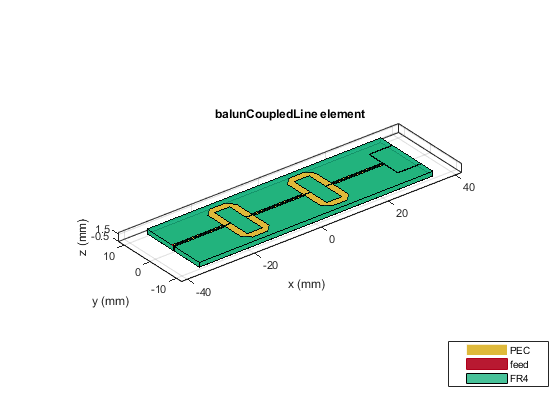Create a coupled-line balun using default properties.

`balun = balunCoupledLine;`

Change the substrate and the dielectric of the balun.

```balun.Substrate = dielectric('Name',{'Teflon','Teflon'},'EpsilonR', ... [2.1 2.1],'LossTangent',[0 0],'Thickness',[0.8e-3 0.8e-3]); balun.Height = 0.8e-3;```

Visualize the balun.

`show(balun)`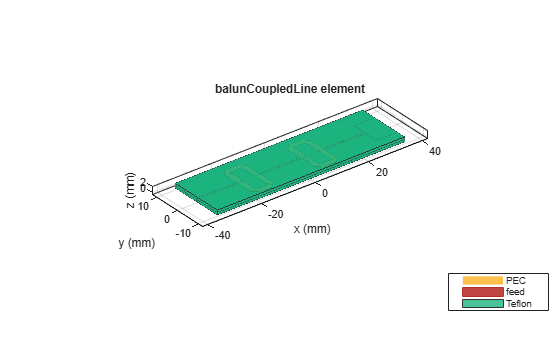Create a coupled-line balun with an `OutputLineSpacing` of 5 mm.

`balun = balunCoupledLine('OutputLineSpacing',0.005);`

Visualize the balun.

`show(balun);`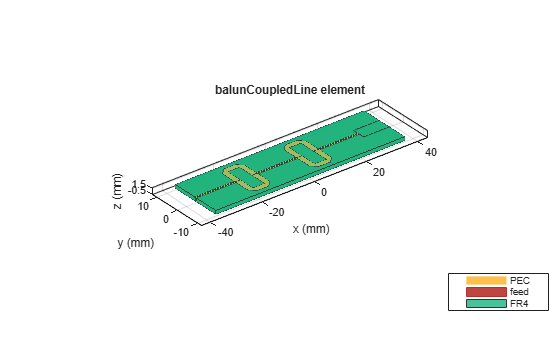Define the frequency at 4 GHz.

`f = 4e9;`

Create a coupled line balun object.

`balun = balunCoupledLine`
```balun = balunCoupledLine with properties: NumCoupledLineSection: 3 CoupledLineLength: 0.0153 CoupledLineWidth: 4.0000e-04 CoupledLineSpacing: 1.4000e-04 UncoupledLineShape: [1×1 ubendMitered] OutputLineLength: 0.0124 OutputLineWidth: 1.5300e-04 OutputLineSpacing: 0.0110 Height: 0.0013 GroundPlaneWidth: 0.0200 Substrate: [1×1 dielectric] Conductor: [1×1 metal] ```
`show(balun)`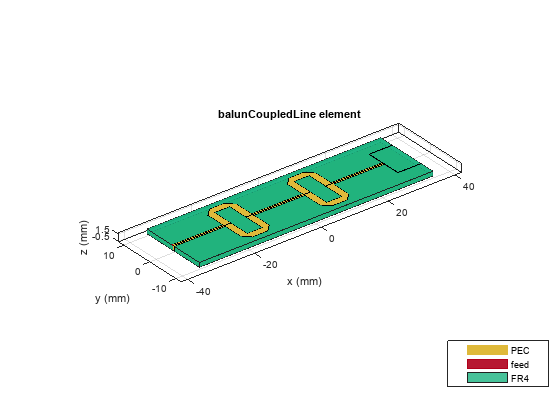Step 1: Design coupled line section

Design the coupled line section of the balun with an even mode impedance of 159 ohms and an odd mode impedance of 51 ohms. Use the helper function designCoupledLine.

`[ClineL,ClineW,ClineS] = designCoupledLine(balun,f,'Z0e',159,'Z0o',51)`
```ClineL = 0.0107 ```
```ClineW = 4.2682e-04 ```
```ClineS = 1.4374e-04 ```

Step 2: Design uncoupled line section

Design the uncoupled line section of the balun with the even and odd mode impedance of 59 ohms. Use the helper function designUncoupledLine.

`[unclineL,unclineW] = designUncoupledLine(balun,f,'Z0',59,'LineLength',0.25)`
```unclineL = 0.0103 ```
```unclineW = 0.0018 ```

Step 3: Design output line section

Design the output line section of the balun at the same frequency to extend the port 2 and port3. Use the helper function designOutputLine.

`[OutL,OutW] = designOutputLine(balun,f,'Z0e',159,'Z0o',51,'Z0',59,'Zref',50)`
```OutL = 0.0109 ```
```OutW = 1.6115e-04 ```

Set all the design dimensions to the coupled balun object.

```balun.CoupledLineLength = ClineL; balun.CoupledLineWidth = ClineW; balun.CoupledLineSpacing = ClineS; UnCoupledLine = ubendMitered; UnCoupledLine.Length = [unclineL/2,unclineL/4,unclineL/2]; UnCoupledLine.Width = [unclineW,unclineW,unclineW]; balun.UncoupledLineShape = UnCoupledLine; balun.OutputLineLength = OutL; balun.OutputLineWidth = OutW; balun.OutputLineSpacing = OutL+ClineS; gndW = 25e-3; balun.GroundPlaneWidth = gndW; show(balun)```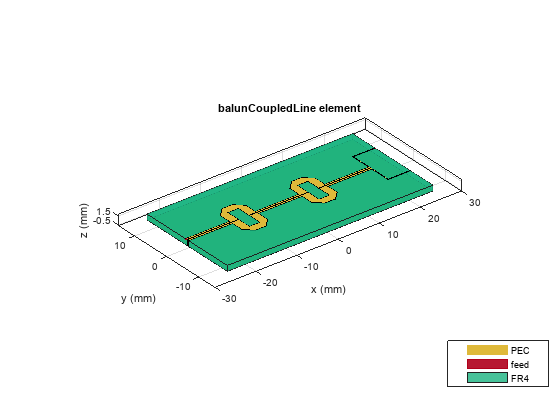Analyze and plot the S-parameters of this balun.

```s11 = sparameters(balun,linspace(3.5e9,4.5e9,31)); figure; rfplot(s11,1,1); hold on; rfplot(s11,1,3) hold on; rfplot(s11,1,2)```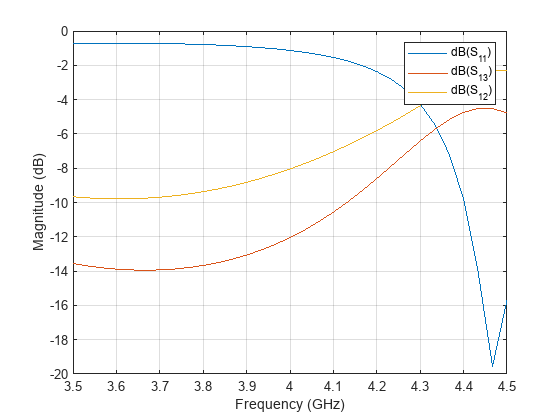Pozar, David M. Microwave Engineering. 4th ed. Hoboken, NJ: Wiley, 2012.